# Section 29Intermezzo 5: The Cost of Computing and Vectors

In section 26.3 we discussed the differences between a structurally recursive program and an equivalent, generative version. The comparison revealed that the generative one is much faster than the structural version. We used both informal arguments, using the number of recursive calls, and measurements, using `time` expressions (exercises 26.3.1 and 26.3.3), to support our conclusion.

While timing the application of a program to specific arguments can help us understand a program's behavior in one situation, it is not a fully convincing argument. After all, applying the same program to some other inputs may require a radically different amount of time. In short, timing programs for specific inputs has the same status as testing programs for specific examples. Just as testing may reveal bugs, timing may reveal anomalies concerning the execution behavior for specific inputs. It does not provide a firm foundation for general statements about the behavior of a program.

This intermezzo introduces a tool for making general statements about the time that programs take to compute a result. The first subsection motivates the tool and illustrates it with several examples, though on an informal basis. The second one provides a rigorous definition. The last one uses the tool to motivate an additional class of Scheme data and some of its basic operations.

## 29.1  Concrete Time, Abstract Time

Let's study the behavior of `how-many`, a function that we understand well:

```(define (how-many a-list)
(cond
[(empty? a-list) 0]
[else (+ (how-many (rest a-list)) 1)]))
```

It consumes a list and computes how many items the list contains.

Here is a sample evaluation:

```  (how-many (list 'a 'b 'c))
```

```= (+ (how-many (list 'b 'c)) 1)
```

```= (+ (+ (how-many (list 'c)) 1) 1)
```

```= (+ (+ (+ (how-many empty) 1) 1) 1)
```

```= 3
```

It consists of only those steps that are natural recursions. The steps in between are always the same. For example, to get from the original application to the first natural recursion, we go through the following steps:

```  (how-many (list 'a 'b 'c))
```

```= (cond
[(empty? (list 'a 'b 'c)) 0]
[else (+ (how-many (rest (list 'a 'b 'c))) 1)])
```

```= (cond
[false 0]
[else (+ (how-many (rest (list 'a 'b 'c))) 1)])
```

```= (cond
[else (+ (how-many (rest (list 'a 'b 'c))) 1)])
```

```= (+ (how-many (rest (list 'a 'b 'c))) 1)
```

The steps between the remaing natural recursions differ only as far as the substitution for `a-list` is concerned.

If we apply `how-many` to a shorter list, we need fewer natural recursion steps:

```  (how-many (list 'e))
= (+ (how-many empty) 1)
= 1
```

If we apply `how-many` to a longer list, we need more natural recursion steps. The number of steps between natural recursions remains the same.

The example suggests that, not surprisingly, the number of evaluation steps depends on the size of the input. More importantly, though, it also implies that the number of natural recrusions is a good measure of the size of an evaluation sequence. After all, we can reconstruct the actual number of steps from this measure and the function definition. For this reason, programmers have come to express the ABSTRACT RUNNING TIME of a program as a relationship between the size of the input and the number of recursion steps in an evaluation.62

In our first example, the size of the input is simply the size of the list. More specifically, if the list contains one item, the evaluation requires one natural recursion. For two items, we recur twice. For a list with N items, the evaluation requires N steps.

Not all functions have such a uniform measure for their abstract running time. Take a look at our first recursive function:

```(define (contains-doll? a-list-of-symbols)
(cond
[(empty? a-list-of-symbols) false]
[else (cond
[(symbol=? (first a-list-of-symbols) 'doll) true]
[else (contains-doll? (rest a-list-of-symbols))])]))
```

If we evaluate

```(contains-doll? (list 'doll 'robot 'ball 'game-boy 'pokemon))
```

the application requires no natural recursion step. In contrast, for the expression

```(contains-doll? (list 'robot 'ball 'game-boy 'pokemon 'doll))
```

the evaluation requires as many natural recursion steps as there are items in the list. Put differently, in the best case, the function can find the answer immediately; in the worst case, the function must search the entire input list.

Programmers cannot assume that inputs are always of the best posisble shape; and they must hope that the inputs are not of the worst possible shape. Instead, they must analyze how much time their functions take on the average. For example, `contains-doll?` may -- on the average -- find `'doll` somewhere in the middle of the list. Thus, we could say that if the input contains N items, the abstract running time of `contains-doll?` is (roughly)-- that is, it naturally recurs half as often as the number of items on the input. Because we already measure the running time of a function in an abstract manner, we can ignore the division by 2. More precisely, we assume that each basic step takes K units of time. If, instead, we use K/2 as the constant, we can calculate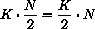which shows that we can ignore other constant factors. To indicate that we are hiding such constants we say that `contains-doll?` takes ``on the order of N steps'' to find `'doll` in a list of N items.

Now consider our standard sorting function from figure 33. Here is a hand-evaluation for a small input:

```  (sort (list 3 1 2))
```

```= (insert 3 (sort (list 1 2)))
```

```= (insert 3 (insert 1 (sort (list 2))))
```

```= (insert 3 (insert 1 (insert 2 (sort empty))))
```

```= (insert 3 (insert 1 (insert 2 empty)))
```

```= (insert 3 (insert 1 (list 2)))
```

```= (insert 3 (cons 2 (insert 1 empty)))
```

```= (insert 3 (list 2 1))
```

```= (insert 3 (list 2 1))
```

```= (list 3 2 1)
```

The evaluation is more complicated than those for `how-many` or `contains-doll?`. It also consists of two phases. During the first one, the natural recursions for `sort` set up as many applications of `insert` as there are items in the list. During the second phase, each application of `insert` traverses a list of 1, 2, 3, ... up to the number of items in the original list (minus one).

Inserting an item is similar to finding an item, so it is not surprising that `insert` behaves like `contains-doll?`. More specifically, the applications of `insert` to a list of N items may trigger N natural recursions or none. On the average, we assume it requires N/2, which means on the order of N. Because there are N applications of `insert`, we have an average of on the order of N2 natural recursions of `insert`.

In summary, if `l` contains N items, evaluating `(sort l)` always requires N natural recursions of `sort` and on the order of N2 natural recursions of `insert`. Taken together, we getsteps, but we will see in exercise 29.2.1 that this is equivalent to saying that insertion sort requires on the order of N2 steps.

Our final example is the function `maxi`:

```;; `maxi : ne-list-of-numbers  ->  number`
;; to determine the maximum of a non-empty list of numbers
(define (maxi alon)
(cond
[(empty? (rest alon)) (first alon)]
[else (cond
[(> (maxi (rest alon)) (first alon)) (maxi (rest alon))]
[else (first alon)])]))
```

In exercise 18.1.12, we investigated its behavior and the behavior of an observationally equivalent function that uses `local`. Here we study its abstract running time rather than just observe some concrete running time.

Let's start with a small example: `(maxi (list 0 1 2 3))`. We know that the result is `3`. Here is the first important step of a hand-evaluation:

```  (maxi (list 0 1 2 3))
= (cond
[(> `(maxi (list 1 2 3))` 0) `(maxi (list 1 2 3))`]
[else 0])
```

From here, we must evaluate the left of the two underlined natural recursions. Because the result is `3` and the condition is thus `true`, we must evaluate the second underlined natural recursion as well.

Focusing on just the natural recursion we see that its hand-evaluation begins with similar steps:

```  (maxi (list 1 2 3))
= (cond
[(> (maxi (list 2 3)) 1) (maxi (list 2 3))]
[else 1])
```

Again, `(maxi (list 2 3))` is evaluated twice because it produces the maximum. Finally, even determining the maximum of `(maxi (list 2 3))` requires two natural recursions:

```  (maxi (list 2 3))
= (cond
[(> (maxi (list 3)) 2) (maxi (list 3))]
[else 2])
```

To summarize, `maxi` requires two natural recursions for each application. The following table counts the instances for our example:

 original expression requires 2 evaluations of `(maxi (list 0 1 2 3))` `(maxi (list 1 2 3))` `(maxi (list 1 2 3))` `(maxi (list 2 3))` `(maxi (list 2 3))` `(maxi (list 3))`
Altogether the hand-evaluation requires eight natural recursions for a list of four items. If we add `4` (or a larger number) at the end of the list, we need to double the number of natural recursions. Thus, in general we need on the order ofrecursions for a list of N numbers when the last number is the maximum.63

While the scenario we considered is the worst possible case, the analysis of `maxi`'s abstract running time explains the phenomenon we studied in exercise 18.1.12. It also explains why a version of `maxi` that uses a local-expression to name the result of the natural recursion is faster:

```;; `maxi2 : ne-list-of-numbers  ->  number`
;; to determine the maximum of a list of numbers
(define (maxi2 alon)
(cond
[(empty? (rest alon)) (first alon)]
[else (local ((define max-of-rest (maxi2 (rest alon))))
(cond
[(> max-of-rest (first alon)) max-of-rest]
[else (first alon)])])))
```

Instead of recomputing the maximum of the rest of the list, this version just refers to the variable twice when the variable stands for the maximum of the rest of the list.

Exercise 29.1.1.   A number tree is either a number or a pair of number trees. Develop the function `sum-tree`, which determines the sum of the numbers in a tree. How should we measure the size of a tree? What is its abstract running time?Solution

Exercise 29.1.2.   Hand-evaluate `(maxi2 (list 0 1 2 3))` in a manner similar to our evaluation of `(maxi (list 0 1 2 3))`. What is the abstract running time of `maxi2`?Solution

## 29.2  The Definition of ``on the Order of''

It is time to introduce a rigorous description of the phrase ``on the order of'' and to explain why it is acceptable to ignore some constants. Any serious programmer must be thoroughly familiar with this notion. It is the most fundamental method for analyzing and comparing the behavior of programs. This intermezzo provides a first glimpse at the idea; a second course on computing usually provides some more in-depth considerations.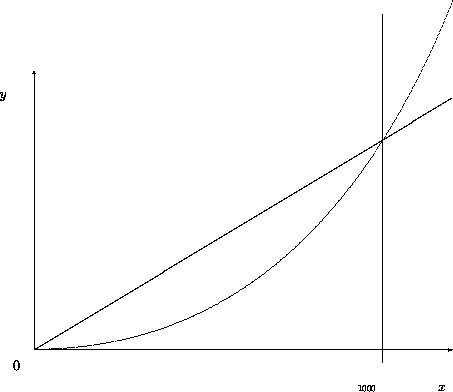Figure 80:  A comparison of two running time expressions

Let's consider a sample ``order of'' claim with concrete examples before we agree on a definition. Recall that a function `F` may require on the order of N steps and a function `G` N2 steps, even though both compute the same results for the same inputs. Now suppose the basic time constants are `1000` for `F` and 1 for `G`. One way to compare the two claims is to tabulate the abstract running time:

 N 1 10 50 100 500 1000 `F` (1000 · N) 1000 10000 50000 100000 500000 1000000 `G` (N · N) 1 100 2500 10000 250000 1000000
At first glance the table seems to say that `G`'s performance is better than `F`'s, because for inputs of the same size (N), `G`'s running time is always smaller than `F`'s. But a closer look reveals that as the inputs get larger, `G`'s advantage decreases. Indeed, for an input of size 1000, the two functions need the same number of steps, and thereafter `G` is always slower than `F`. Figure 80 compares the graphs of the two expressions. It shows that the linear graph for 1000 · N dominates the curve of N · N for some finite number of points but thereafter it is below the curve.

The concrete example recalls two important facts about our informal discussion of abstract running time. First, our abstract description is always a claim about the relationship between two quantities: the size of the input and the number of natural recursions evaluated. More precisely, the relationship is a (mathematical) function that maps an abstract size measure of the input to an abstract measure of the running time. Second, when we compare ``on the order of'' properties of functions, such aswe really mean to compare the corresponding functions that consume N and produce the above results. In short, a statement concerning the order of things compares two functions on natural numbers (`N`).

The comparison of functions on `N` is difficult because they are infinite. If a function f produces larger outputs than some other function g for all natural numbers, then f is clearly larger than g. But what if this comparison fails for just a few inputs? Or for 1,000 such as the one illustrated in figure 80? Because we would still like to make approximate judgments, programmers and scientists adapt the mathematical notion of comparing functions up to some factor and some finite number of exceptions.

ORDER-OF (BIG-O): Given a function g on the natural numbers, O(g) (pronounced: ``big-O of g'') is a class of functions on natural numbers. A function f is in O(g) if there exist numbers c and bigEnough such that for all n > bigEnough, it is true that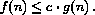Recall the performance of F and G above. For the first, we assumed that it consumed time according to the following function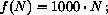the performance of second one obeyed the function g:Using the definition of big-O, we can say that f is O(g), because for all n > 1000,which means bigEnough = 1000 and c = 1.

More important, the definition of big-O provides us with a shorthand for stating claims about a function's running time. For example, from now on, we say `how-many`'s running time is O(N). Keep in mind that N is the standard abbreviation of the (mathematical) function g(N) = N. Similarly, we can say that, in the worst case, `sort`'s running time is O(N2) and `maxi`'s is O(2N).

Finally, the definition of big-O explains why we don't have to pay attention to specific constants in our comparsions of abstract running time. Consider `maxi` and `maxi2`. We know that `maxi`'s worst-case running time is in O(2N), `maxi2`'s is in O(N). Say, we need the maximum of a list with 10 numbers. Assuming `maxi` and `maxi2` roughly consume the same amount of time per basic step, `maxi` will need 210 = 1024 steps and `maxi2` will need 10 steps, which means `maxi2` will be faster. Now even if `maxi2`'s basic step requires twice as much time as `maxi`'s basic step, `maxi2` is still around 50 times faster. Futhermore, if we double the size of the input list, `maxi`'s apparent disadvantage totally disappears. In general, the larger the input is, the less relevant are the specific constants.

Exercise 29.2.1.   In the first subsection, we stated that the function f(n) = n2 + n belongs to the class O(n2). Determine the pair of numbers c and bigEnough that verify this claim.Solution

Exercise 29.2.2.   Consider the functions f(n) = 2n and g(n) = 1000 · n. Show that g belongs to O(f), which means that f is abstractly speaking more (or at least equally) expensive than g. If the input size is guaranteed to be between 3 and 12, which function is better?Solution

Exercise 29.2.3.   Compare f(n) = n log n and g(n) = n2. Does f belong to O(g) and/or g to O(f)?Solution

## 29.3  A First Look at Vectors

Until now we have paid little attention to how much time it takes to retrieve data from structures or lists. Now that we have a tool for stating general judgments, let's take a close look at this basic computation step. Recall the last problem of the preceding part: finding a route in a graph. The program `find-route` requires two auxiliaries: `find-route/list` and `neighbors`. We paid a lot of attention to `find-route/list` and none to `neighbors`. Indeed, developing `neighbors` was just an exercise (see 28.1.2), because looking up a value in a list is by now a routine programming task.

Here is a possible definition for `neighbors`:

```;; `neighbors : node graph  ->  (listof node)`
;; to lookup the `node` in `graph`
(define (neighbors node graph)
(cond
[(empty? graph) (error 'neighbors "can't happen")]
[else (cond
[(symbol=? (first (first graph)) node) (second (first graph))]
[else (neighbors node (rest graph))])]))
```

The function is similar to `contains-doll?` and has roughly the same behavior. More concretely, `neighbors` is O(N) when we assume that `graph` is a list of N nodes.

Considering that `neighbors` is used at every stage of the evaluation of `find-route`, `neighbors` is possibly a bottleneck. As a matter of fact, if the route we are looking for involves `N` nodes (the maximum), `neighbors` is applied N times, so the algorithm requires O(N2) steps in `neighbors`.

In contrast to lists, structures deal with value extractions as a constant time operation. At first glance this observation seems to suggest that we use structures as representations of graphs. A closer look, however, shows that this idea doesn't work easily. The graph algorithm works best if we are able to work with the names of nodes and access a node's neighbors based on the name. A name could be a symbol or the node's number in the graph. In general, what we really wish to have in a programming language is

a class of compound values size with constant lookup time,
based on ``keys.''
Because the problem is so common, Scheme and most other languages offer at least one built-in solution.

Here we study the class of vectors. A vector is a well-defined mathematical class of data with specific basic operations. For our purposes, it suffices to know how to construct them, how to extract values, and how to recognize them:

1. The operation `vector` is like `list`. It consumes an arbitrary number of values and creates a compound value from them: a vector. For example, `(vector V-0 ... V-n)` creates a vector from `V-0` through `V-n`.

2. DrScheme also provides a vector analogue to `build-list`. It is called `build-vector`. Here is how it works:

```(build-vector N f) = (vector (f 0) ... (f (- N 1)))
```

That is, `build-vector` consumes a natural number `N` and a function `f` on natural numbers. It then builds a vector of `N` items by applying `f` to `0`, ..., `N-1`.

3. The operation `vector-ref` extracts a value from a vector in constant time, that is, for `i` between `0` and `n` (inclusive):

```(vector-ref (vector V-0 ... V-n) i) = V-i
```

In short, extracting values from a vector is O(1).

If `vector-ref` is applied to a vector and a natural number that is smaller than `0` or larger than `n`, `vector-ref` signals an error.

4. The operation `vector-length` produces the number of items in a vector:

```(vector-length (vector V-0 ... V-n)) = (+ n 1)
```

5. The operation `vector?` is the vector-predicate:

```(vector? (vector V-0 ... V-n)) = true
(vector? U) = false
```

if `U` is a value that isn't created with `vector`.

We can think of vectors as functions on a small, finite range of natural numbers. Their range is the full class of Scheme values. We can also think of them as tables that associate a small, finite range of natural numbers with Scheme values. Using vectors we can represent graphs like those in figures 76 and 78 if we use numbers as names. For example:

 A B C D E F G 0 1 2 3 4 5 6
Using this translation, we can also produce a vector-based representation of the graph in figure 76:
 ```(define Graph-as-list '((A (B E)) (B (E F)) (C (D)) (D ()) (E (C F)) (F (D G)) (G ()))) ``` ```(define Graph-as-vector (vector (list 1 4) (list 4 5) (list 3) empty (list 2 5) (list 3 6) empty)) ```
The definition on the left is the original list-based representation; the one on the right is a vector representation. The vector's `i`-th field contains the list of neighbors of the `i`-th node.

The data definitions for `node` and `graph` change in the expected manner. Let's assume that N is the number of nodes in the given graph:

A node is an natural number between 0 and N - 1.

A graph is a vector of `node`s: ```(vectorof (listof node))```.

The notation `(vectorof X)` is similar to ```(listof X)```. It denotes a vector that contains items from some undetermined class of data `X`.

Now we can redefine `neighbors`:

```;; `neighbors : node graph  ->  (listof node)`
;; to lookup the `node` in `graph`
(define (neighbors node graph)
(vector-ref graph node))
```

As a result, looking up the neighbors of a node becomes a constant-time operation, and we can truly ignore it when we study the abstract running time of `find-route`.

Exercise 29.3.1.   Test the new `neighbors` function. Use the strategy of section 17.8 to formulate the tests as boolean expressions.Solution

Exercise 29.3.2.   Adapt the rest of the `find-route` program to the new vector representation. Adapt the tests from exercises 28.1.3 through 28.1.5 to check the new program.

Measure how much time the two `find-route` programs consume to compute a route from node A to node E in the graph of figure 76. Recall that `(time expr)` measures how long it takes to evaluate `expr`. It is good practice to evaluate `expr`, say, 1000 times when we measure time. This produces more accurate measurements.Solution

Exercise 29.3.3.   Translate the cyclic graph from figure 78 into our vector representation of graphs.Solution

Before we can truly program with vectors, we must understand the data definition. The situation is comparable to that when we first encountered lists. We know that `vector`, like `cons`, is provided by Scheme, but we don't have a data definition that directs our program development efforts.

So, let us take a look at vectors. Roughly speaking, `vector` is like `cons`. The `cons` primitive constructs lists, the `vector` primitive creates vectors. Since programming with lists usually means programming with the selectors `first` and `rest`, programming with vectors must mean programming with `vector-ref`. Unlike `first` and `rest`, however, `vector-ref` requires manipulating the vector and an index into a vector. This suggests that programming with vectors really means thinking about indices, which are natural numbers.

Let's look at some simple examples to confirm this abstract judgment. Here is the first one:

```;; `vector-sum-for-3 : (vector number number number)  ->  number`
(define (vector-sum-for-3 v)
(+ (vector-ref v 0)
(vector-ref v 1)
(vector-ref v 2)))
```

The function `vector-sum-for-3` consumes vectors of three numbers and produces their sum. It uses `vector-ref` to extract the three numbers and adds them up. What varies in the three selector expressions is the index; the vector remains the same.

Consider a second, more interesting example: `vector-sum`, a generalization of `vector-sum-for-3`. It consumes an arbitrarily large vector of numbers and produces the sum of the numbers:

```;; `vector-sum : (vectorof number)  ->  number`
;; to sum up the numbers in `v`
(define (vector-sum v) ...)
```

Here are some examples:

```(= (vector-sum (vector -1 3/4 1/4))
0)

(= (vector-sum (vector .1 .1 .1 .1 .1 .1 .1 .1 .1 .1))
1)

(= (vector-sum (vector))
0)
```

The last example suggests that we want a reasonable answer even if the vector is empty. As with `empty`, we use `0` as the answer in this case.

The problem is that the one natural number associated with `v`, its length, is not an argument of `vector-sum`. The length of `v` is of course just an indication of how many items in `v` are to be processed, which in turn refers to legal indices of `v`. This reasoning forces us to develop an auxiliary function that consumes the vector and a natural number:

```;; `vector-sum-aux : (vectorof number) N  ->  number`
;; to sum up the numbers in `v` relative to `i`
(define (vector-sum-aux v i) ...)
```

The natural choice for the initial value of `i` is the length of `v`, which suggests the following completion of `vector-sum`:

```(define (vector-sum v)
(vector-sum-aux v (vector-length v)))
```

Based on this definition, we can also adapt the examples for `vector-sum` to `vector-sum-aux`:

```(= (vector-sum-aux (vector -1 3/4 1/4) 3)
0)

(= (vector-sum-aux (vector .1 .1 .1 .1 .1 .1 .1 .1 .1 .1) 10)
1)

(= (vector-sum-aux (vector) 0)
0)
```

Unfortunately, this doesn't clarify the role of the second argument. To do that, we need to proceed to the next stage of the design process: template development.

When we develop templates for functions of two arguments, we must first decide which of the arguments must be processed, that is, which of the two will vary in the course of a computation. The `vector-sum-for-3` example suggests that it is the second argument in this case. Because this argument belongs to the class of natural numbers, we follow the design recipe for those:

```(define (vector-sum-aux v i)
(cond
[(zero? i) ...]
[else ... (vector-sum-aux v (sub1 i)) ...]))
```

Although we considered `i` to be the length of the vector initially, the template suggests that we should consider it the number of items of `v` that `vector-sum-aux` must consider and thus as an index into `v`.

The elaboration of `i`'s use naturally leads to a better purpose statement for `vector-sum-aux`:

```;; `vector-sum-aux : (vectorof number) N  ->  number`
;; to sum up the numbers in `v` with index in [`0`, `i`)
(define (vector-sum-aux v i)
(cond
[(zero? i) ...]
[else ... (vector-sum-aux v (sub1 i)) ...]))
```

Excluding `i` is natural because it is initially `(vector-length v)` and thus not an index.

 ```;; `vector-sum : (vectorof number)  ->  number` ;; to compute the sum of the numbers in `v` (define (vector-sum v) (vector-sum-aux v (vector-length v))) ``` ```;; `vector-sum-aux : (vectorof number) N  ->  number` ;; to sum the numbers in `v` with index in [`0`, `i`) (define (vector-sum-aux v i) (cond [(zero? i) 0] [else (+ (vector-ref v (sub1 i)) (vector-sum-aux v (sub1 i)))])) ``` Figure 81:  Summing up the numbers in a vector (version 1) ```;; `lr-vector-sum : (vectorof number)  ->  number` ;; to sum up the numbers in `v` (define (lr-vector-sum v) (vector-sum-aux v 0)) ;; `vector-sum : (vectorof number)  ->  number` ;; to sum up the numbers in `v` with index in [`i`, `(vector-length v)`) (define (vector-sum-aux v i) (cond [(= i (vector-length v)) 0] [else (+ (vector-ref v i) (vector-sum-aux v (add1 i)))])) ``` Figure 82:  Summing up the numbers in a vector (version 2)

To transform the template into a complete function definition, we consider each clause of the `cond`:

1. If `i` is `0`, there are no further items to be considered because there are no vector fields between `0` and `i` with `i` excluded. Therefore the result is `0`.

2. Otherwise, `(vector-sum-aux v (sub1 i))` computes the sum of the numbers in `v` between `0` and `(sub1 i)` [exclusive]. This leaves out the vector field with index ```(sub1 i)```, which according to the purpose statement must be included. By adding `(vector-ref v (sub1 i))`, we get the desired result:

```(+ (vector-ref v (sub1 i)) (vector-sum-aux v (sub1 i)))
```

See figure 81 for the complete program.

If we were to evaluate one of the examples for `vector-sum-aux` by hand, we would see that it extracts the numbers from the vector in a right to left order as `i` decreases to `0`. A natural question is whether we can invert this order. In other words: is there a function that extracts the numbers in a left to right order?

The answer is to develop a function that processes the class of natural numbers below `(vector-length v)` and to start at the first feasible index: `0`. Developing this function is just another instance of the design recipe for variants of natural numbers from section 11.4. The new function definition is shown in figure 82. The new auxiliary function now consumes `0` and counts up to `(vector-length v)`. A hand-evaluation of

```(lr-vector-sum (vector 0 1 2 3))
```

shows that `vector-sum-aux` indeed extracts the items from `v` from left to right.

The definition of `lr-vector-sum` shows why we need to study alternative definitions of classes of natural numbers. Sometimes it is necessary to count down to `0`. But at other times it is equally useful, and natural, to count from `0` up to some other number.

The two functions also show how important it is to reason about intervals. The auxiliary vector-processing functions process intervals of the given vector. A good purpose statement specifies the exact interval that the function works on. Indeed, once we understand the exact interval specification, formulating the full function is relatively straightforward. We will see the importance of this point when we return to the study of vector-processing functions in the last section.

Exercise 29.3.4.   Evaluate `(vector-sum-aux (vector -1 3/4 1/4) 3)` by hand. Show the major steps only. Check the evaluation with DrScheme's stepper. In what order does the function add up the numbers of the vector?

Use a local-expression to define a single function `vector-sum`. Then remove the vector argument from the inner function definition. Why can we do that?Solution

Exercise 29.3.5.   Evaluate `(lr-vector-sum (vector -1 3/4 1/4))` by hand. Show the major steps only. Check the evaluation with DrScheme's stepper. In what order does the function add up the numbers of the vector?

Use a local-expression to define a single function `lr-vector-sum`. Then remove those arguments from the inner function definition that remain the same during an evaluation. Also introduce definitions for those expressions that always evaluate to the same value during the evaluation. Why is this useful?Solution

Exercise 29.3.6.   The list-based analogue of `vector-sum` is `list-sum`:

```;; `list-sum : (listof number)  ->  number`
;; to compute the sum of the numbers on `alon`
(define (list-sum alon)
(list-sum-aux alon (length alon)))

;; `list-sum-aux : N (listof number)  ->  number`
;; to compute the sum of the first `L` numbers on `alon`
(define (list-sum-aux L alon)
(cond
[(zero? L) 0]
[else (+ (list-ref alon (sub1 L)) (list-sum-aux (sub1 L) alon))]))
```

Instead of using the structural definition of the list, the developer of this program used the size of the list -- a natural number -- as the guiding element in the design process.

The resulting definition uses Scheme's `list-ref` function to access each item on the list. Looking up an item in a list with `list-ref` is an O(N) operation for lists of N items. Determine the abstract running time of `sum` (from section 9.5), `vector-sum-aux` and `list-sum-aux`. What does this suggest about program development?Solution

Exercise 29.3.7.   Develop the function `norm`, which consumes a vector of numbers and produces the square root of the sum of the squares of its numbers. Another name for `norm` is `distance-to-0`, because the result is the distance of a vector to the origin, when we interpret the vector as a point.Solution

Exercise 29.3.8.   Develop the function `vector-contains-doll?`. It consumes a vector of symbols and determines whether the vector contains the symbol `'doll`. If so, it produces the index of `'doll`'s field; otherwise, it produces `false`.

Determine the abstract running time of `vector-contains-doll?` and compare with that of `contains-doll?`, which we discussed in the preceding subsection.

Now discuss the following problem. Suppose we are to represent a collection of symbols. The only interesting problem concerning the collection is to determine whether it contains some given symbol. Which data representation is preferable for the collection: lists or vectors? Why?Solution

Exercise 29.3.9.   Develop the function `binary-contains?`. It consumes a sorted vector of numbers and a key, which is also a number. The goal is to determine the index of the key, if it occurs in the vector, or `false`. Use the binary-search algorithm from section 27.3.

Determine the abstract running time of `binary-contains?` and compare with that of `contains?`, the function that searches for a key in a vector in the linear fashion of `vector-contains-doll?`.

Suppose we are to represent a collection of numbers. The only interesting problem concerning the collection is to determine whether it contains some given number. Which data representation is preferable for the collection: lists or vectors? Why?Solution

Exercise 29.3.10.   Develop the function `vector-count`. It consumes a vector `v` of symbols and a symbol `s`. Its result is the number of `s` that occur in `v`.

Determine the abstract running time of `vector-count` and compare with that of `count`, which counts how many times `s` occurs in a list of symbols.

Suppose we are to represent a collection of symbols. The only interesting problem concerning the collection is to determine how many times it contains some given symbol. Which data representation is preferable for the collection: lists or vectors? Why? What do exercises 29.3.8, 29.3.9, and this exercise suggest?Solution

While accessing the items of a vector is one kind of programming problem, constructing vectors is an entirely different problem. When we know the number of items in a vector, we can construct it using `vector`. When we we wish to write programs that work on a large class of vectors independent of their size, however, we need `build-vector`.

Consider the following simple example. Suppose we represent the velocity of an object with a vector. For example, `(vector 1 2)` represents the velocity of an object on a plane that moves `1` unit to the right and `2` down in each time unit. For comparison, ```(vector -1 2 1)``` is the veloicity of an object in space; it moves `-6` units in the x direction in 6 time units, `12` units in the y direction in 6 time units, and `6` units in the z direction in 6 time units. We call ```(vector -6 12 6)``` the displacement of the object in 6 time units.

Let's develop a function that computes the displacement for an object with some velocity `v` in `t` time units:

```;; `displacement : (vectorof number) number  ->  (vectorof number)`
;; to compute the displacement of `v` and `t`
(define (displacement v t) ...)
```

Computing the displacement is straightforward for some examples:

```(equal? (displacement (vector 1 2) 3)
(vector 3 6))

(equal? (displacement (vector -1 2 1) 6)
(vector -6 12 6))

(equal? (displacement (vector -1 -2) 2)
(vector -2 -4))
```

We just multiply each component of the object with the number, which yields a new vector.

The examples' meaning for our programming problem is that `displacement` must construct a vector of the same length as `v` and must use the items in `v` to compute those of the new vectors. Here is how we build a vector of the same how-many as some given vector `v`:

```(build-vector (vector-length v) ...)
```

Now we need to replace ... with a function that computes the `0`-th, `1`-st, and so on items of the new vector:

```;; `new-item :  N  ->  number`
;; to compute the contents of the new vector at the `i`-th position
(define (new-item index) ...)
```

Following our discussion, we multiply `(vector-ref v i)` with `t` and that's all.

Take a look at the complete definition:

```;; `displacement : (vectorof number) number  ->  (vectorof number)`
;; to compute the displacement of `v` and `t`
(define (displacement v t)
(local ((define (new-item i) (* (vector-ref v i) t)))
(build-vector (vector-length v) new-item)))
```

The locally defined function is not recursive. We can thus replace it with a plain lambda-expression:

```;; `displacement : (vectorof number) number  ->  (vectorof number)`
;; to compute the displacement of `v` and `t`
(define (displacement v t)
(build-vector (vector-length v) (lambda (i) (* (vector-ref v i) t))))
```

Mathematicians call this function scalar product. They have also studied many other operations on vectors, and in Scheme we can develop those in a natural manner.

Exercise 29.3.11.   Develop the function `id-vector`, which consumes a natural number and produces a vector of that many `1`'s.Solution

Exercise 29.3.12.   Develop the functions `vector+` and `vector - `, which compute the pointwise sum and differences of two vectors. That is, each consumes two vectors and produces a vector by manipulating corresponding programs. Assume the given vectors are of the same length. Also develop the functions `checked-vector+` and `checked-vector - `.Solution

Exercise 29.3.13.   Develop the function `distance`, which consumes two vectors and computes their distance. Think of the distance of two vectors as the length of the line between them.Solution

Exercise 29.3.14.   Develop a vector representation for chessboards of size n × n for n in `N`. Then develop the following two functions on chessboards:

```;; `build-board : N (N N  ->  boolean)  ->  board`
;; to create a board of size `n` x `n`,
;; fill each position with indices `i` and `j` with `(f i j)`
(define (build-board n f) ...)

;; `board-ref : board N N  ->  boolean`
;; to access a position with indices `i`, `j` on `a-board`
(define (board-ref a-board i j) ...)
```

Can we now run the program of section 28.2 using vectors instead of lists? Inspect the solution of exercises 28.2.3 and 28.2.4.Solution

Exercise 29.3.15.   A matrix is a chessboard of numbers. Use the chessboard representation of exercise 29.3.14 to represent the matrix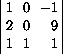Using `build-board`, develop the function `transpose`, which creates a mirror image of the matrix along its diagonal from the upper-left corner to the lower-right one. For example, the given matrix turns into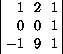More generally, the item at (i,j) becomes the item at (j,i).Solution

62 We speak of an abstract running time because the measure ignores the details of how much time primitive steps take and how much time the overall evaluation takes.

63 More precisely, the evaluation consists of 2N-1 steps, but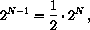which shows that we ignore a (small) constant when we say on the order of 2N.